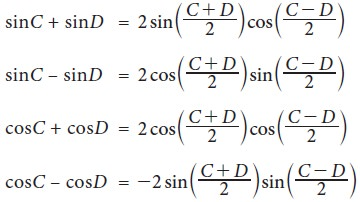Home | | Business Maths 11th std | Transformation formulae

# Transformation formulae

The two sets of transformation formulae are described below. i. Transformation of the products into sum or difference ii. Transformation of sum or difference into product

Transformation formulae

The two sets of transformation formulae are described below.

## 1. Transformation of the products  into sum or difference## 2. Transformation of sum or difference into productTags : Solved Example Problems, Exercise | Mathematics , 11th Business Mathematics and Statistics(EMS) : Chapter 4 : Trigonometry
Study Material, Lecturing Notes, Assignment, Reference, Wiki description explanation, brief detail
11th Business Mathematics and Statistics(EMS) : Chapter 4 : Trigonometry : Transformation formulae | Solved Example Problems, Exercise | Mathematics